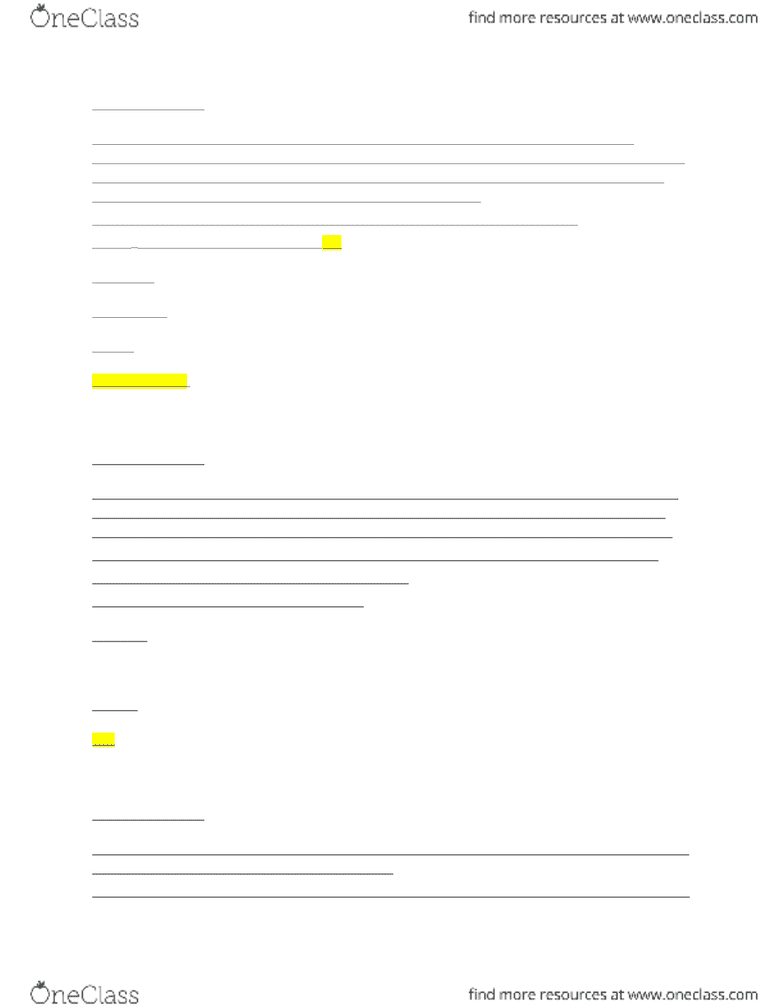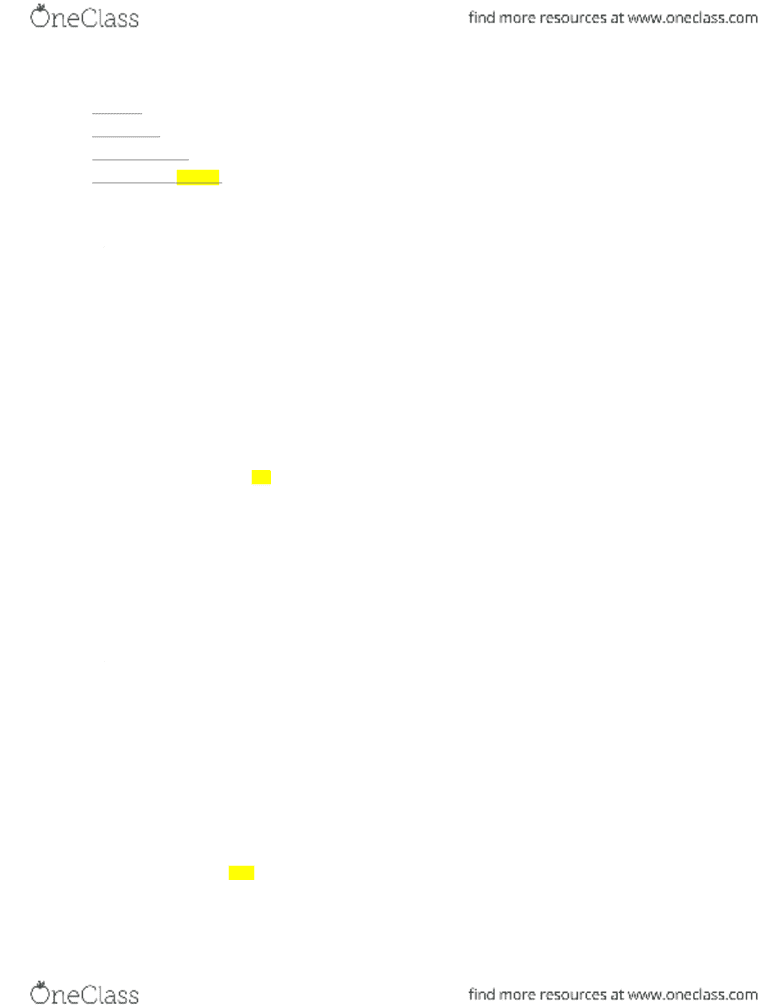Class Notes (1,100,000)
CA (620,000)
UW (20,000)
CHEM (300)
CHEM120 (40)
Lecture 5

# CHEM120 Lecture Notes - Lecture 5: Calorimeter, International System Of Units, Joule

Department
Chemistry
Course Code
CHEM120
Professor
Richard Marta
Lecture
5

This preview shows pages 1-2. to view the full 6 pages of the document.Question 1: (1 point)
A 0.005637-mol sample of an organic compound was burned in oxygen in a bomb calorimeter. The
temperature of the calorimeter increased from 24.9 o
C to 28.8 o
C. If the heat capacity of the calorimeter is 4.90
kJ ( o
C) -1
, then what is the constant volume heat of combustion of this compound, in kilojoules per mole ?
(Remember to include a "+" or "-" sign in your answer, as appropriate.)
Enter your answer with 3 significant figures. Do not include units as part of your answer.
C=q/t 4.90 /3.90 *0.005637 = 222.88 = 223
4.90kj*3.90
0.005637 mol
3390.10
-3.39x10 3 kJ/mol
Question 2: (1 point)
Brass has a density of 8.40 g/cm 3
and a specific heat of 0.385 J g -1
o
C -1
. A 12.6 cm 3
piece of brass at an initial
temperature of 153 o
C is dropped into an insulated container with 186.9 grams of water initially at 24.4 o
C.
What will be the final temperature of the brass-water mixture? (The specific heat of water is 4.184 J g -1
o
C -1
.)
Enter your answer with 3 significant figures. Enter only numbers in your answer. (Do not include units.)
105.84 * 0.385 J(Tf – 153) + 186.9 g (Tf – 24.4) * 4.184 J = 0
40.74844t – 6234.5052 + 781.9896t - 19080.546 = 0
822.4146t
=0.0326
30.8
Question 3: (1 point)
What is w when a gas is compressed from 41.7 L to 23.7 L using a constant external pressure of 803 mmHg?
(Remember to include a "+" or "-" sign as appropriate.)
Enter your answer in acceptable SI units, accurate to 3 significant figures. Use an acceptable SI abbreviation

Only pages 1-2 are available for preview. Some parts have been intentionally blurred.for units!
W=-Pext (V)
= 107.0578 * (-18)
= -1927.041 J = -1.93 kJ
Question 4: (1 point)
What is w when 3.23 kg of H2O(l), initially at 25.0oC, is converted into water vapour at 119oC against a constant
external pressure of 1.00 atm? Assume that the vapour behaves ideally and that the density of liquid water is 1.00
g/mL. (Remember to include a "+" or "-" sign as appropriate.)
Enter your answer in acceptable SI units, accurate to 3 significant figures. Use an acceptable SI abbreviation
for units!
W = -P (V)) = -584239.2003 = -584
= -101.325 * (vf-vi) = 5765.9926
Vf = nRT/P =(179.285079)(0.082058)(392.15) / 1.00 = 5769.222601L
Vi= 3.23 kg mL * 1000g = 3230ml / 1000ml = 3.23 L
1 g
Question 5: (1 point)
What is the internal energy change for the system if 527 joules of heat flows into the system from the surroundings
and 523 joules of work is done by the system on the surroundings?
Enter an integer, with a "+" or "-" sign as appropriate. Don't forget to include an appropriate SI abbreviation
for the units!
U = q + w = (527) + (-523) = 4 J
###### You're Reading a Preview

Unlock to view full version